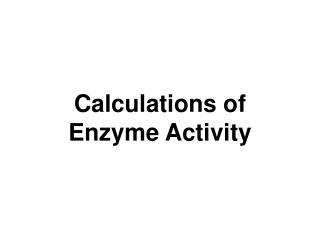Download PresentationCalculations of Enzyme Activity

# Calculations of Enzyme Activity - PowerPoint PPT Presentation

Calculations of Enzyme Activity. Enzyme Activity. Unit of enzyme activity: Used to measure total units of activity in a given volume of solution. Specific activity: Used to follow the increasing purity of an enzyme through several procedural steps. Molecular activity:I am the owner, or an agent authorized to act on behalf of the owner, of the copyrighted work described.
Download Presentation## Calculations of Enzyme Activity

An Image/Link below is provided (as is) to download presentation

Download Policy: Content on the Website is provided to you AS IS for your information and personal use and may not be sold / licensed / shared on other websites without getting consent from its author.While downloading, if for some reason you are not able to download a presentation, the publisher may have deleted the file from their server.

- - - - - - - - - - - - - - - - - - - - - - - - - - E N D - - - - - - - - - - - - - - - - - - - - - - - - - -
Presentation Transcript
1. Calculations ofEnzyme Activity

2. Enzyme Activity Unit of enzyme activity: Used to measure total units of activity in a given volume of solution. Specific activity: Used to follow the increasing purity of an enzyme through several procedural steps. Molecular activity: Used to compare activities of different enzymes. Also called the turn-over number (TON = kcat)

3. Enzyme Activity Classical units: Unit of enzyme activity: mmol substrate transformed/min = unit Specific activity: mmol substrate/min-mg E = unit/mg E Molecular activity: mmol substrate/min- mmol E = units/mmol E

4. Enzyme Activity New international units: Unit of enzyme activity: mol substrate/sec = katal Specific activity: mol substrate/sec-kg E = katal/kg E Molecular activity: mol substrate/sec-mol E = katal/mol E

5. Example 1 The rate of an enzyme catalyzed reaction is 35 μmol/min at [S] = 10-4 M, (KM = 2 x 10-5). Calculate the velocity at [S] = 2 x 10-6 M. Work the problem.

6. Example 1 Answer The rate of an enzyme catalyzed reaction is 35 μmol/min at [S] = 10-4 M, (KM = 2 x 10-5). Calculate the velocity at [S] = 2 x 10-6 M. First calculate VM using the Michaelis-Menton eqn: VM [S] VM (10-4) VM (10-4) v = -----------, so: 35 = ------------------ = -------------- KM + [S] 2 x 10-5 + 10-4 1.2 x 10-4 VM = 1.2(35) = 42 mmol/min; then calculate v: 42 (2 x 10-6) 84 x 10-6 v = ------------------------ = ------------ = 3.8 mmol/min 2 x 10-5 + 2 x 10-6 22 x 10-6

7. Example 2 An enzyme (1.84 μgm, MW = 36800) catalyzes a reaction in presence of excess substrate at a rate of 4.2 μmol substrate/min. What is the TON in min-1 ? What is the TON in sec-1 ? Work the problem.

8. Example 2 Answer An enzyme (1.84 μgm, MW = 36800) catalyzes a reaction in presence of excess substrate at a rate of 4.2 μmol substrate/min. What is the TON ? 1.84 μgm μ mol E = ------------------------- = 5 x 10-5μmol E 36800μgm/μmol 4.2μmol/min TON = ------------------ = 84000 min-1 5 x 10-5μmol

9. Example 2 Answer What is the value of this TON (84000 min-1) in units of sec-1 ? 84000 min-1 1 sec-1 TON E = ------------------ x ---------- = 1400sec-1 60 min-1

10. Example 3 Ten micrograms of carbonic anhydrase (MW = 30000) in the presence of excess substrate exhibits a reaction rate of 6.82 x 103μmol/min. At [S] = 0.012 M the rate is 3.41 x 103μmol/min. a. What is Vm ? b. What is KM ? c. What is k2 (kcat) ? Work these.

11. Example 3 • The rate in presence of excess substrate is Vmax • so: • Vmax = 6.86 x 103μmol/min. • b. At [S] = 0.012 M the rate is 3.41 x 103μmol/min which is ½ Vmax so: • KM = 0.012 M. • This may also be determined using the • Michaelis-Menton equation. • c. Divide Vmax by μmol of ET to find kcat. • kcat = 2.05 x 107 min-1

12. End of Enzyme Activity